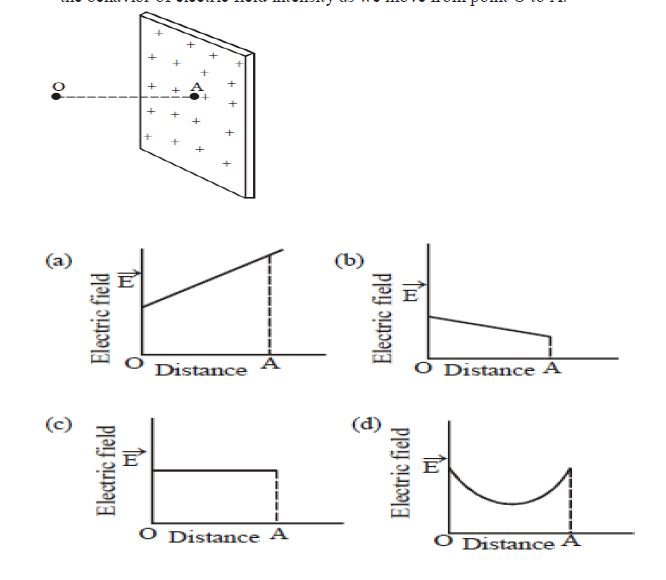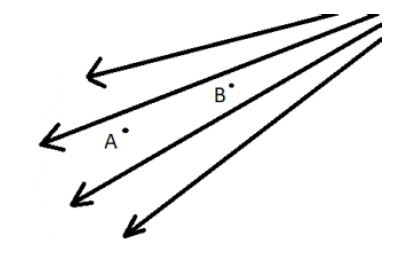# MCQs Electric Charges and Field Chapter-1 Physics Class 12

## Important MCQs Electric Charges and Field Chapter-1 Physics Class 12

In this section you will learn some important multiple choice , case study , assertion reason questions with answer from Class 12 Physics Chapter 1.

### Electric Charges and Fields MCQs

1. A force of 4N is acting between two charges in air. If the space between them is completely filled with glass (relative permittivity = 8), then the new force will be

a) 2N

b) 5N

c) 0.2N

d) 0.5N

2. A charge q is placed at the center of the line joining two equal charges Q. The system of three charges will be in equilibrium if q is equal to

a) –Q/2

b) –Q/4

c) Q/4

d) Q/2

3. Two point charges Q and -3Q are placed some distance apart. If the electric field at the location of Q is E, the field at the location of -3Q is

a) E

b) -E

c) E/3

d) –E/3

4. A soap bubble is given a negative charge, then its radius

a) Decreases

b) Increases

c) Remains unchanged

d) Nothing can be predicted as information is insufficient

5. Two equally charged identical metal spheres A and B repel each other with a force F. Another identical uncharged sphere C is touched to A and then placed midway between A and B. The net force on C is in the direction

a) F towards A

b) F towards B

c) 2F towards A

d) 2F towards B

6. An electric dipole when placed in a uniform electric field will have minimum potential energy, if the angle between dipole moment and electric field is

a) Zero

b) π/ 2

c) π

d) π/3

7. An electric dipole consists of two opposite charges of magnitude 1μC separated by a distance of 2cm. The dipole is placed in an electric filed 10-5 V/m. The maximum torque experienced by the dipole is

a) 10-3 Nm

b) 2 x10-13   Nm

c) 3 x10-3 Nm

d) 4x 10-3 Nm

8. Electric field lines provide information about

(a) Field strength

(b) direction

(c) nature of charge

(d) all of these

9. There are two charges + 1mc and + 2mc kept at certain separation. The ratio of electro static forces acting on them will be in the ratio of

a) 1 : 2

b) 2 : 1

c) 1 : 1

d) 1 : 4

10. Electric field on the axis of a small electric dipole at a distance r is E1 rand E2 at a distance of 2r, on a line of perpendicular bisector. Then, ( E1 / E2 ) is

a) 2

b) 4

c) 8

d) 16

### Electric Charges and Fields MCQs  Source based questions:

Smallest charge that can exist in nature is the charge of an electron. During friction it is only the transfer of electron which makes the body charged. Hence net charge on a body is an integral multiple of charge of an electron (1.6 x 10-19 C) i.e., q = ±ne where r= 1, 2, 3, 4 …. Hence no body can have a charge represented as 1.8e, 2.7e, 2e/5, etc. Recently, it has been discovered that elementary particles such as protons or neutrons are elemental units called quarks.

11. Which of the following properties is not satisfied by an electric charge?

(a) Total charge conservation.

(b) Quantization of charge.

(c) Two types of charge.

(d) Circular line of force.

12. Which one of the following charges is possible?

(a) 5.8 x 10-18 C

(b) 3.2 x 10-18 C

(c) 4.5 x 10-19 C

(d) 8.6 x 10-19 C

13. If a charge on a body is 1nC, then how many electrons are present on the body?

(a) 6.25 x 1027

(b) 1.6 x 1019

(c) 6.25 X 1028

(d) 6.25 X 109

### Electric Charges and Fields MCQs Case study questions:

Gauss’s law for the electric field describes the static electric field generated by a distribution of electric charges. It states that the electric flux through any closed surface is proportional to the total electric charge enclosed by this surface. By convention, a positive electric charge generates a positive electric field. The law was published posthumously in 1867 as part of a collection of work by the famous German mathematician Carl Friedrich Gauss.

∮E.ds = q /∈0

14. Gauss law cannot be used to find which of the following quantity?

a) Electric field intensity

b) Electric flux density

c) Charge

d) Permittivity

15. Select the correct statements from the following.

I. The electric field due to a charge outside the Gaussian surface contributes zero net flux through the surface.

II. Total flux linked with a closed body, not enclosing any charge will be zero.

III. Total electric flux, if a dipole is enclosed by a surface is zero.

(a) I and II

(b) II and III

(c) I and III

(d) I, II and III

16. Figure shows the part of an infinite plane sheet of charge. Which of the following graphs correctly shows the behavior of electric field intensity as we move from point O to A.### Case study questions:

17. In Gauss Theorem, Number of electric field lines entering the surface is __________ to the field lines leaving the surface.

A. greater

B. less

C. equal

D. two times.

18. A plane surface is rotated in a uniform electric field. When is the flux of the electric field through the surface maximum?

a. When the surface is perpendicular to the field

b. When the surface is parallel to the field

c. When the surface is at an angle of 300 with the field

d. When the surface is at an angle of 450 with the field

19. What is the electric flux through a cube, which encloses an electric dipole?

a) q/ε0

b) q/6ε0

c) q/4πε0

d) Zero

20. A point charge q is placed at a distance a/2 directly above the center of the square of side a. The electric flux through the square is

a. q/ε0

b. q/6ε0

c. q/4πε0

d. q/2ε0

21. Which of the following statements is not true about Gauss’s law?

(a) Gauss’s law is true for any closed surface.

(b) The term q on the right side of Gauss’s law includes the sum of all charges enclosed by the surface.

(c) Gauss’s law is not much useful in calculating electrostatic field when the system has some symmetry.

(d) Gauss’s law is based on the inverse square dependence on distance contained in the coulomb’s law.

22 . Two unlike charges separated by a distance of 1m attract each other with a force of 0.108N. If the charges are in the ratio 1:3, the weak charge is

a) 2μC

b) 4μC

c) 6μC

d) 5μC

23. if the electric field is given by (6i + 3j + 4k) N/c, the flux through a surface of area 20m2 in the Y-Z plane is

a) 12 units

b) 120 units

c) 360 units

d) 80 units

24. If the electric flux entering and leaving an enclosed surface respectively is Φ1 and Φ2, the electric charge inside the surface will be

(a) (Φ2 + Φ2) × εo

(b) (Φ2 – Φ2) × εo

(c) (Φ1 + Φ2) × εo

(d) (Φ2 – Φ1) × εo

25. A cylinder of radius R and length L is placed in a uniform electric field E parallel to the axis of the cylinder. The total flux over the curved surface of the cylinder is

(a) zero

(b) πR2E

(c) 2πR2E

(d) E / πR2

26. Consider the lines of force as shown in the figure. Two unit positive charges are kept at points A and B. Which of the following is correct?a) Charge at A will suffer greater force

b) Charge at B will suffer greater force

c) Force at both points will be same but non-zero

d) Force at both points will be the same

### Assertion-Reason Questions:

DIRECTION: Read the two statements Assertion (A) and Reason (R) carefully to mark the correct option out of the options given below:

27. Assertion: No two electric lines of force can intersect each other.

Reason: Tangent at any point of electric line of force gives the direction of electric field.

(a) Assertion and Reason both are correct statements and Reason is correct explanation for Assertion.

(b) Assertion and Reason both are correct statements but Reason is not correct explanation for Assertion.

(c) Assertion is correct statement but Reason is wrong statement.

(d) Assertion is wrong statement but Reason is correct statement

28. Assertion: Coulombs law of force is applicable for point charges at rest.

Reason: Coulombs law is a central force.

(a) Assertion and Reason both are correct statements and Reason is correct explanation for Assertion.

(b) Assertion and Reason both are correct statements but Reason is not correct explanation for Assertion.

(c) Assertion is correct statement but Reason is wrong statement.

(d) Assertion is wrong statement but Reason is correct statement

29. Assertion: Electric charge is quantized.

Reason: Charging is because of transfer of an integral number of protons or electrons.

(a) Assertion and Reason both are correct statements and Reason is correct explanation for Assertion.

(b) Assertion and Reason both are correct statements but Reason is not correct explanation for Assertion.

(c) Assertion is correct statement but Reason is wrong statement.

(d) Assertion is wrong statement but Reason is correct statement

30. Assertion: If there exists coulomb attraction between two bodies, both of them may not be charged.

Reason: In coulomb attraction two bodies are oppositely charged.

(a) Assertion and Reason both are correct statements and Reason is correct explanation for Assertion.

(b) Assertion and Reason both are correct statements but Reason is not correct explanation for Assertion.

(c) Assertion is correct statement but Reason is wrong statement.

(d) Assertion is wrong statement but Reason is correct statement

Source: Kendriya Vidyalaya Sangathan, Hyderabad Region